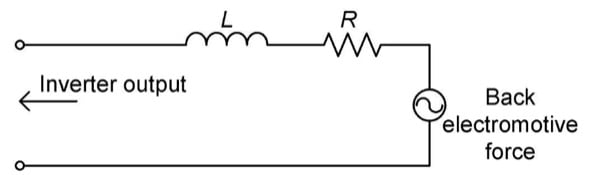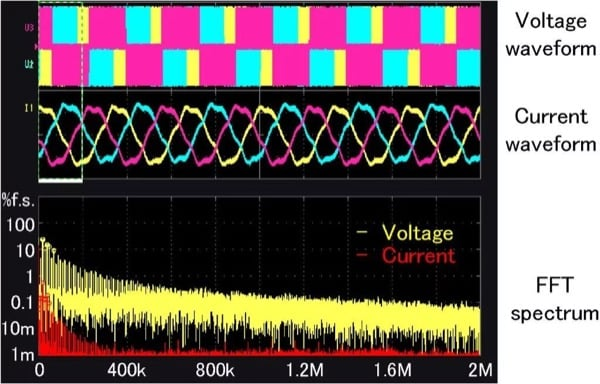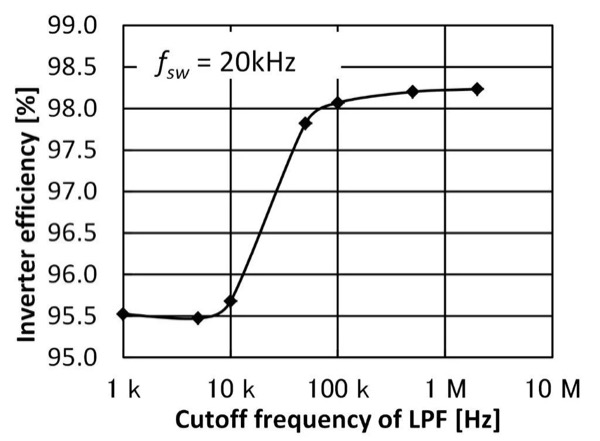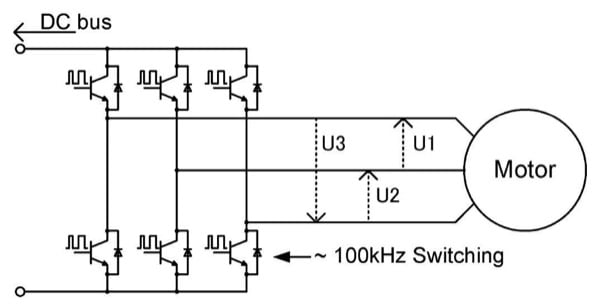# High-Precision Power Measurement of SiC Inverters

## Introduction

Development of higher-efficiency, more compact motor drive systems is a key priority for manufacturers of EVs and HEVs as well as the rail industry, among other sectors of the economy, where companies have started using SiC power semiconductors in order to boost the efficiency and shrink the size of the inverters that are principal components of motor drive systems. Expected advantages of SiC power semiconductors include smaller passive component dimensions thanks to higher switching frequencies and lower-loss performance thanks to low on-resistance values. Accurate power measurement is a critical precondition for evaluating motor drive systems, but power measurement of SiC inverters requires high-precision measurement across a broader band of frequencies than in the past. This paper introduces a range of topics including expertise related to power, efficiency, and loss measurement of SiC inverters and motor drive systems, along with actual measurement results.Figure 1: Measuring the efficiency of a motor drive system

## Measuring the Efficiency of Inverters and Motors

During evaluation of motor drive systems that incorporate inverters and motors, it is possible to measure efficiency and loss by measuring the inverter’s input and output power and the motor’s power and then calculating the ratio or differential between the input and output values. Fig. 1 provides a measurement block diagram illustrating the measurement of the efficiency of a standard motor drive system.

The output of inverters and motors fluctuates over time. Consequently, accurate measurement is made difficult by imperfect synchronization of measurement timing and by differences in calculation methods when calculating efficiency and loss by measuring the respective points with separate instruments. Accordingly, it is necessary to take all measurements simultaneously, either by using a single instrument for all of them or through the synchronized control of multiple instruments. This requirement can be met by using a power analyzer. Standard power analyzers provide four to six channels of power measurement along with motor analysis functionality, allowing them to measure efficiency and loss with a high degree of precision.

Looking more closely at the measurement process, results vary depending on how the time period across which power calculations are performed is defined. Power analyzers determine the periods across which calculations are performed by detecting zero-cross events in input waveforms. Generally speaking, the channel corresponding to the signal for which zero-cross events will be detected can be set as desired as the synchronization source. Setting the optimal synchronization source enables stable power measurement, making it possible to measure efficiency and loss with a high degree of precision. For example, if the inverter is fed DC input, the calculation periods can be synchronized by setting the same synchronization source for the input and output channels. In this way, it is possible to measure efficiency and loss in a stable manner. In the example shown in Figure 1, power at two points and motor power at one point are being measured in a stable manner by setting the synchronization source for all channels to the inverter’s output current.

## Measuring an Inverter’s Input Power

To measure efficiency and loss, it is necessary to measure the power being input to the inverter. This input power will serve as the basis for measuring efficiency and loss. Generally speaking, either DC or AC commercial power is used as inverter input. If the values yielded by measurement of the input and output power contain an error component, it will have a significant effect on the efficiency and loss values. Consequently, it is necessary to measure the inverter’s input power with a high degree of precision. For example, an error of 0.5% in the input power measured value for an inverter with an efficiency of 99% will result in an error of 50% for the loss. Although it is possible to calculate power using a general-purpose waveform recorder, one must exercise caution to ensure that a sufficient level of accuracy has been defined for the band that you wish to measure.

Caution is especially warranted during DC power measurement, which should be preceded by adjusting the power analyzer and current sensors’ DC offsets. If the power analyzer provides a zero-adjustment function, perform zero-adjustment after zeroing out input to the power analyzer and current sensors. In this way, it is possible enable accurate DC measurement by canceling out the instrument’s DC offset.

# Measuring an Inverter’s Output Power

Inverters generate PWM-modulated output that includes the switching frequency and its harmonic components. Consequently, power measurement must be performed over a wider band than when measuring DC or commercial frequency power.

Let’s study the band that is needed in order to measure power at the switching frequency and its harmonics. Figure 2 provides an equivalent circuit for a motor that is driven by an inverter. Since the motor’s windings have an inductance component, high-frequency current is less likely to flow to the motor. Since the voltage is a PWM waveform, it can be approximated as a rectangular wave. At this time, the current will take the shape of a triangular waveform. When calculating RMS values for the triangular waveform over the frequency domain, measurement can yield RMS values with an error of 0.1% or less if harmonics can be measured to the 5th order. Here the active power Pf can be expressed as a function of the voltage Uf, the current If, and the voltage-current phase difference θf as follows:

P_f=U_f*I_f*cos(θ_f)

Consequently, if either the voltage or current is 0, the active power for that frequency component will be 0. Assuming measurement at a precision of 0.1%, current at 7th order and higher harmonic components can be ignored, as noted above. Therefore, the ability to measure voltage, current, and phase difference accurately within the band of 5 times to 7 times the switching frequency is sufficient in order to measure power at the switching frequency and its harmonics with an error of 0.1% or less. However, loss in an actual motor includes the magnetic material’s core loss as well as losses from factors such as wire skin effects in addition to the resistance portion shown in Figure 2. Consequently, a somewhat wider frequency band is needed in order to more accurately measure power at the switching frequency and its harmonics. The band that is actually needed is affected by factors such as the frequency characteristics of the respective losses.

Figure 3 shows the actual voltage and current waveforms of a motor driven by an SiC inverter, as well as associated FFT results. Table 1 provides detailed information about the measurement targets. Since the voltage is a PWM waveform, an examination of the FFT results reveals frequency components in excess of 1 MHz. Standard power analyzers do not provide a sufficient measurement band to measure voltage waveforms with the required degree of accuracy. Looking at the current, it is apparent that the current components do not exceed about 200 kHz. In addition, the waveform closely resembles a sine wave. This shape derives from the fact that the motor’s inductance component makes it less likely that high-frequency current will flow, as described above.

In this way, it is desirable to use a power analyzer with favorable characteristics for voltage, current, and phase difference characteristics in the frequency band of at least 5 to 7 times the switching frequency in order to allow accurate measurement of the inverter’s output power. Use of increasingly high switching frequencies for SiC inverters has the effect of requiring a higher-frequency band in this regard.Figure 2: Equivalent circuit for a motor (1 phase)Figure 3: Waveforms and FFT results for an actual inverter-driven motor (measured with the Power Analyzer PW6001)Table 1: Specifications of measured SiC inverter and motor

Generally speaking, current sensors are used when measuring current in a motor drive system. In such applications, the current sensors’ phase error becomes problematic. All current sensors exhibit a tendency toward increased phase error at higher frequencies, and this tendency becomes a source of error when measuring high-frequency power. As shown in Figure 2, the motor windings’ inductance component is dominant at high frequencies. As a result, power at the switching frequency and its harmonics is characterized by a lower power factor. Based on Equation (1), phase error has an extremely large impact on power measurement error at low power factor values ( values of approximately 90°). Consequently, it is not possible to measure power at a high degree of precision unless the current sensors’ phase error can be corrected. Hioki’s Power Analyzer PW6001 provides functionality for compensation for current sensor phase error, as shown in Figure 4. This phase compensation function makes it possible to measure inverter output power more accurately.Figure 4: Compensating a current sensor’s phase error

## Measuring a Motor’s Power

In order to measure the overall efficiency and loss of a motor or motor drive system, it is necessary to measure the motor’s power. To calculate motor power using Equation (2), we all need to measure torque and rpm.

P_m = T*2*π*n/60

Pm [W]: Motor power
T [N·m]: Torque
n [rpm]: Motor rpm

The motor’s rpm is measured using a tachometer or pulse encoder, while torque is measured using a torque meter. In order to measure efficiency and loss, it is necessary to measure power and motor power at the same time. Consequently, we need to use a power analyzer that can accept signals from a tachometer, pulse encoder, and torque meter as input.

### Example Measurement of the Efficiency of an Inverter with SiC Power Semiconductor

Figure 5 illustrates the results of measuring the efficiency of an SiC inverter that is driving a motor. The setup uses a Hioki Power Analyzer PW6001 and Current Box PW9100, and the figure illustrates the results of measurement while varying the cutoff frequency of the PW6001’s LPF from 1 kHz to 2 MHz. The measurement targets are the same as those described in Table 1. The measured efficiency values change dramatically around a cutoff frequency of 10 kHz to 50 kHz. This change reflects the difference in whether power at the switching frequency and its harmonic components are being measured. In short, efficiency values at and below 10 kHz derive from measurement of only the power at the fundamental frequency, which is synchronized with the motor’s rpm, and its harmonic components. On the other hand, efficiency values at and above 50 kHz derive from also the measurement of power at the switching frequency and its harmonic components. At and above 50 kHz, efficiency values increase as the cutoff frequency increases. This change is a result of the ability to measure the higher-order harmonic components of the switching frequency.

In this way, the PW6001 Power Analyzer is capable of high-precision, high-stability measurement of motor drive system efficiency and loss up to the 2 MHz band, indicating that the instrument can measure efficiency and loss based on accurate measurement of power at the switching frequency and its harmonic components.Figure 5: Efficiency measurement results of an SiC inverter while varying the Power Analyzer PW6001’s LPF cutoff frequency

## Effects of Common-Mode Voltage

Figure 6 provides a voltage wiring schematic describing measurement of the output power of a 3-phase/3-wire inverter. Since the power analyzer will measure line voltage, a large common-mode voltage will be applied across its channels. In addition, this common-mode voltage includes switching frequency and associated harmonic components. Consequently, it is necessary to make measurements with a power analyzer that has a high common-mode rejection ratio (CMRR) for high frequencies. A CMRR of 80 dB has an effect of 0.01% of the common-mode voltage on the displayed values. In other words, if a common-mode voltage of 100 V is input, there would be an effect of 0.01 V on display values.

Figure 7 illustrates the results of measuring the line voltage and common-mode voltage of an SiC inverter. The FFT results are similar to the results shown in Fig. 3, making it clear that the common-mode voltage includes switching frequency and associated harmonic components. Consequently, it can be concluded that as the frequency of the switching frequency increases, so does that of the common-mode voltage. Inverters that use SiC power semiconductors are being designed with increasingly high switching frequencies. As a result, it is desirable to choose a power analyzer with a high CMRR for higher frequencies.Figure 6: Wiring connections when measuring inverter output power (3P3W3M)Figure 7: Common-mode voltage of inverter output voltage

## Countermeasures for Current Sensor Noise

When measuring a motor or inverter with a high rated capacity, it is necessary to measure large currents on the order of several hundred amperes. It is standard practice to use current sensors when measuring large currents. Inverters produce large amounts of noise, and it is essential to implement measures to address the effects of noise on the sensors themselves and on the route along which the current sensors’ output signals are transmitted in order to ensure accurate power measurement. Hioki offers a line of high-precision current sensors with such features for use with power analyzers. Consequently, it is possible to perform power measurement in a manner that is highly resistant to noise simply by connecting the power analyzer and current sensors with a dedicated connector.

## Power Analyzer Frequency Band and Sampling Frequency

Figure 8 illustrates the relationship between sampling frequency and analog band in a typical power analyzer. The analog band of most power analyzers’ input circuitry is greater than half the sampling frequency fs (i.e., fs / 2). In such instruments, the voltage and current components that exist in frequencies higher than (fs / 2) appear in the low-frequency domain as folding noise. This phenomenon is generally known as aliasing.

When measuring targets that include frequency components across a broad band like a PWM waveform, it becomes impossible to distinguish between the folding noise and the actual signal. The result of this phenomenon is additional measurement error and reduced repeatability in power measurement. Moreover, it becomes impossible to distinguish between the folding noise and actual harmonics in harmonic analysis. The result is that accurate analysis becomes impossible, and, for example, detection of false harmonic components more likely.

As shown in Figure 3, inverter output voltage includes components in excess of 1 MHz. Standard power analyzers have sampling frequencies ranging from 100 kHz to about 5 MHz. Consequently, there are voltage components at frequencies in excess of (fs / 2). In such cases, accurate measurement is not possible when the analog band and sampling frequency are related as shown in Figure 8. To enable accurate measurement, it is necessary to limit the analog band to less than (fs / 2). In other words, the band that can actually be used is less than half the sampling frequency.

In this way, when measuring and analyzing inverter output power, it is necessary to use a measuring instrument that has been designed in accord with sampling principles. Hioki’s power analyzers are designed in this way. For example, the Power Analyzer PW6001 has a sampling frequency of 5 MHz, versus an analog band of 2 MHz/-3 dB. Consequently, the instrument is capable of simultaneous broadband power measurement, accurate harmonic analysis, and accurate FFT analysis.Figure 8: Relationship between analog band and sampling frequency in a standard power analyzer

## Summary

This article has introduced key considerations that come into play when measuring the efficiency and loss of inverters and motors while offering actual measurement examples, as well as related topics such as requirements for measuring instruments used in such applications. It has devoted special attention to considerations that apply when measuring SiC inverters, which have been entering into increasingly widespread use in recent years, as compared to conventional inverters. We also presented actual measurement results to demonstrate how the efficiency and loss of SiC inverters can be measured with a high degree of precision and stability by eliminating various sources of error. It is the author’s hope that the discussions will serve as a useful guide in power, efficiency, and loss measurement of SiC inverters and motor drive systems.

Kazunobu Hayashi works as a Lead Engineer at Hioki E.E. Corporation, a Japanese electrical measuring equipment developer and manufacturer based in Ueda, City, Nagano Prefecture. The company was first established in June 1935, and registered as Hioki E.E. on January 5th, 1952

### References

1. Thal, E., K. Masuda, and E. Wiesner, “New 800A/1200V Full SiC Module” Bodo’s Power Systems, April 2015, pp.28-31.
2. Fuji Electronic, “Joint Development of Converter-Inverter for The Tokaido Shinkansen Cars Using SiC Power Semiconductor Modules” http://www.fujielectric.com/company/ news/2015/20150625120019879.html
3. Mitsubishi Electric, “Mitsubishi Electric’s Railcar Traction Inverter with All-SiC Power Modules Achieves 40% Power Savings” http:// www.mitsubishielectric.com/news/2015/0622-a_print.html
4. Yoda, H., H. Kobayashi, and S. Takiguchi, “Current Measurement Methods that Deliver High Precision Power Analysis in the Field of Power Electronics” Bodo’s Power Systems, April 2016, pp.38-42.
5. Ikeda, K., and H. Masuda, “High-Precision, Wideband, Highly Stable Current Sensing Technology” Bodo’s Power Systems, July 2016, pp.22-28.